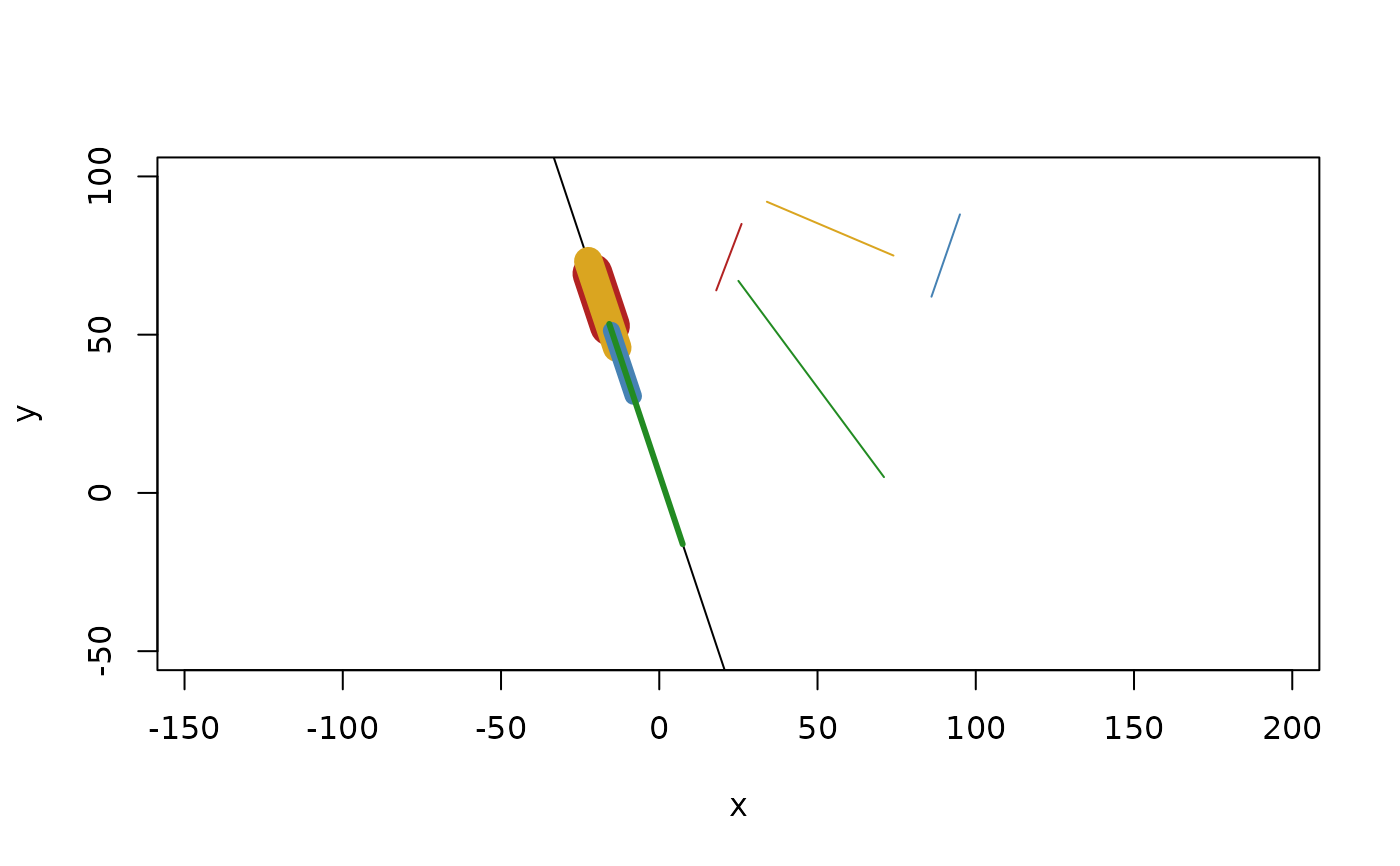Projecting geometries to lines and planes transforms the geometries to lie on the given line or plane by movement orthogonal to the line or plane. The geometry of the result of the projection is the same as the input. Not all geometries can be projected to lines and planes. Specifically, iso rectangles and iso cubes cannot do either and only 3 dimensional geometries can be projected to a plane. Circles and spheres are special in the sense that no ellipse and ellipsoid primitives exist. Because of this, projections will retain the radius and circularity of the primitives despite this not being geometrically sound. Projecting a 3D circle to a line will keep the orientation of the circle, while projecting it to a plane will adopt the orientation of the plane.

## Usage

project(x, target, ...)

# S3 method for euclid_geometry
project(x, target, ...)

## Arguments

x

A vector of geometries to project or map

target

A vector of lines or planes

...

Arguments passed on to methods

## Value

A vector of geometries of the same class as x

Other Projections: map_to(), normal()

## Examples

# Project a collection of segments to a line
p <- point(sample(100, 8), sample(100, 8))
s <- segment(p[1:4], p[5:8])

project(s, line(3, 1, -6))
#> <2D segments >
#>  [<x:-21.1, y:69.3>, <x:-15.6, y:52.8>]
#>  [<x:-13.3, y:45.9>, <x:-22.4, y:73.2>]
#>  [<x:-8.2, y:30.6>, <x:-15.1, y:51.3>]
#>  [<x:-15.8, y:53.4>, <x:7.4, y:-16.2>]

plot(line(3, 1, -6), xlim = c(-50, 100), ylim = c(-50, 100))
euclid_plot(s, col = c("firebrick", "goldenrod", "steelblue", "forestgreen"))
euclid_plot(
project(s, line(3, 1, -6)),
col = c("firebrick", "goldenrod", "steelblue", "forestgreen"),
lwd = seq(20, 3, length.out = 4)
)# Project a line to the x plane
project(line(point(3, 7, -3), point(-2, 6, 0)),
plane(point(0, 0, 0), vec(1, 0, 0)))
#> <3D lines >
#>  <x:0, y:7, z:-3, dx:0, dy:-1, dz:3>